# Long Multiplication

In this mini-lesson, we will learn about long multiplication by understanding the multiplication of 2 digits numbers, the column method of multiplication, and how to apply them while solving problems. We will also discover interesting facts around them.

We are dealing in multiplications of numbers from very long times.

Consider that James wants to plant saplings in his backyard, such that he can plant 190 saplings in a row.And he wants to make 150 such rows, how many saplings he will be going to require?

In this case, we have to perform multiplication of 190 and 150 the result will be the number of saplings required.

Let's explore long multiplication in this session.

## What Is Long Multiplication?

Consider that we have to multiply any two numbers greater than 10 or 100, we usually perform long multiplication.

Let's take an example $$31 \times 49$$

Here, we are multiplying 31 with 49, but if we write any one of these numbers in expanded form for example 31

$\text{31} = \text{30} + \text{1}$

30 is the tenth part and 1 is the unit part.

So $$31 \times 49$$ can be written as $$30 \times 49 + 1 \times 49$$

First, we are multiplying 49 with 30 and then we are multiplying 49 with 1, and then we are adding them.

So, instead of direct multiplication, we have performed long multiplication, which makes the process simple and accurate.

## How Do You Do Long Multiplication?

Let's take an example

### Example

Multiply: $$31 \times 49$$

### Solution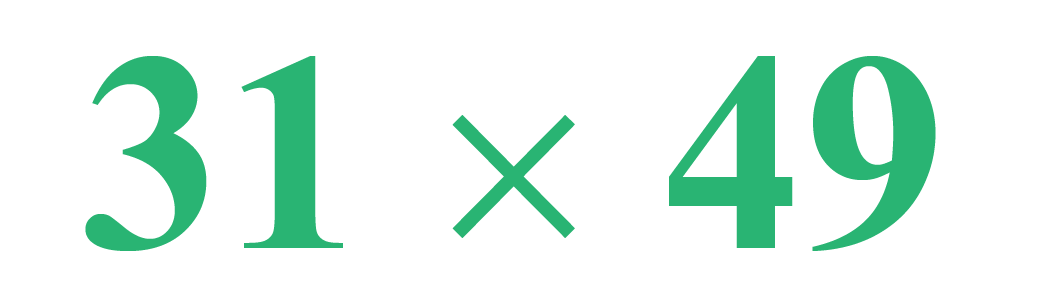Step 1: First multiply 49 with 1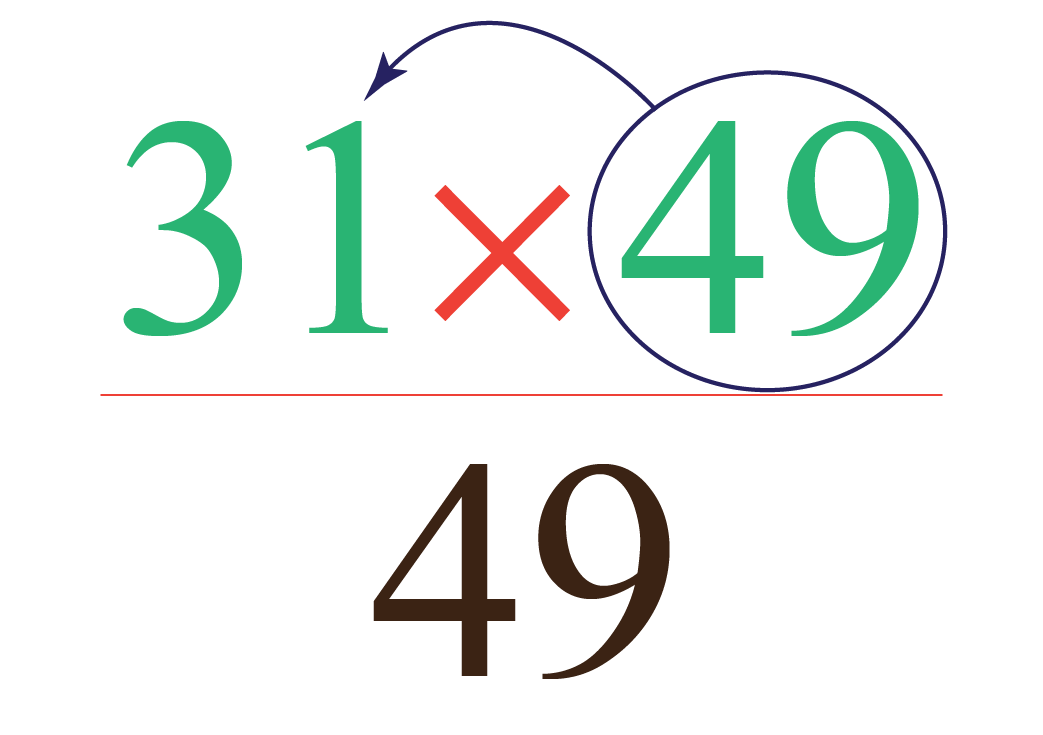Step 2: Now, multiply 49 with 3, and put a cross sign just below 9 (the unit place of the number 49), this cross sign represents a 0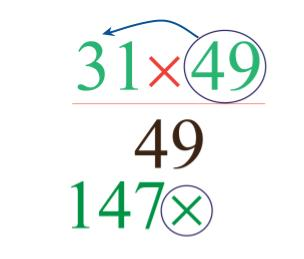Step 3: Write zeros before the number 49 so that it will cover till the number 1 of 147, write the numbers just below another number so that the addition becomes easy.

Step 4: Add these two numbers 0049 and 1470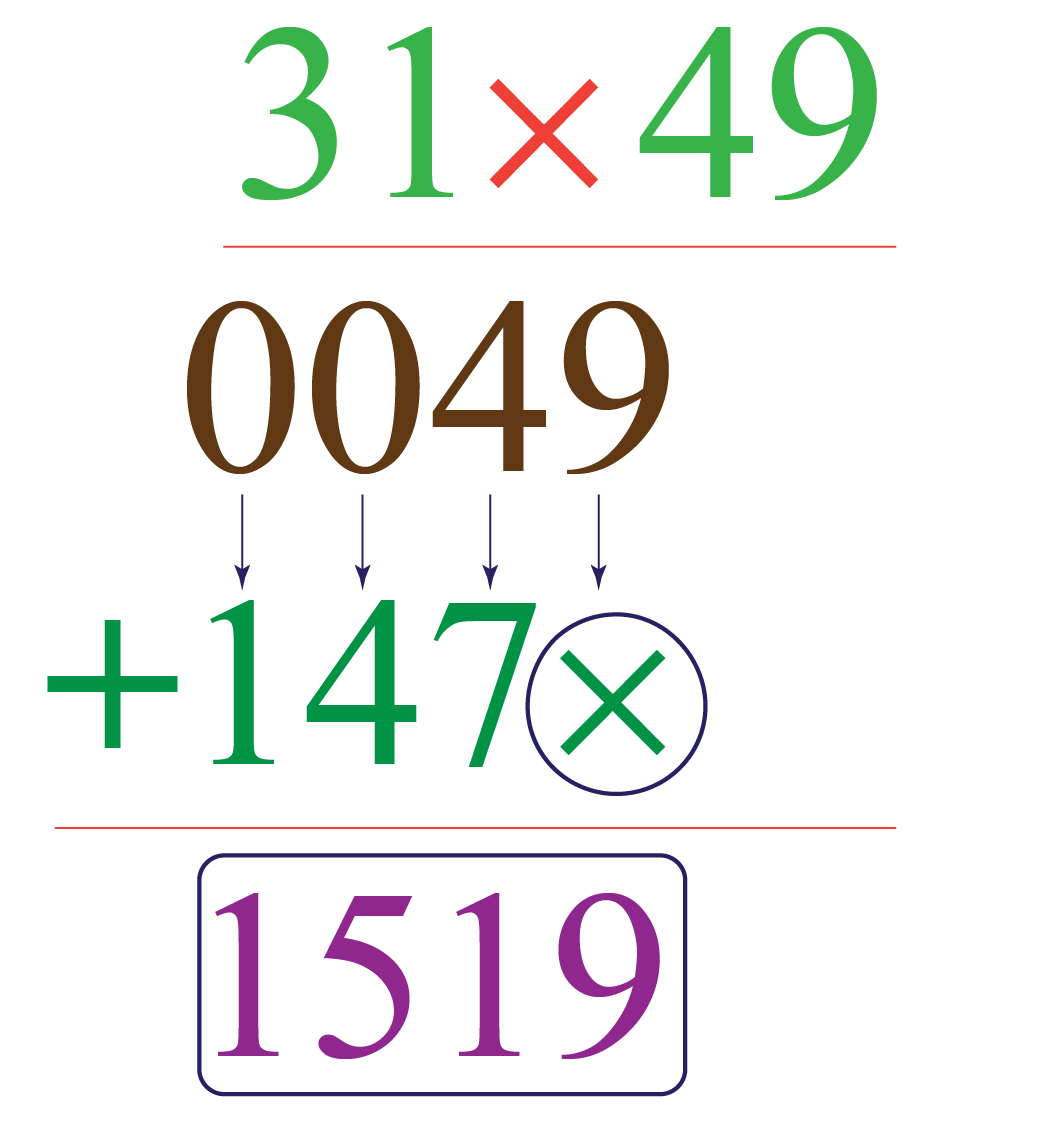So, $$31 \times 49 = 1519$$

## How do You do Long Multiplication With Two Digits?

Let's take a few examples of long multiplication with two and three digits.

### Example

Multiply: $$81 \times 17$$

### Solution

While performing the multiplication, keep the smaller number to the right-hand side.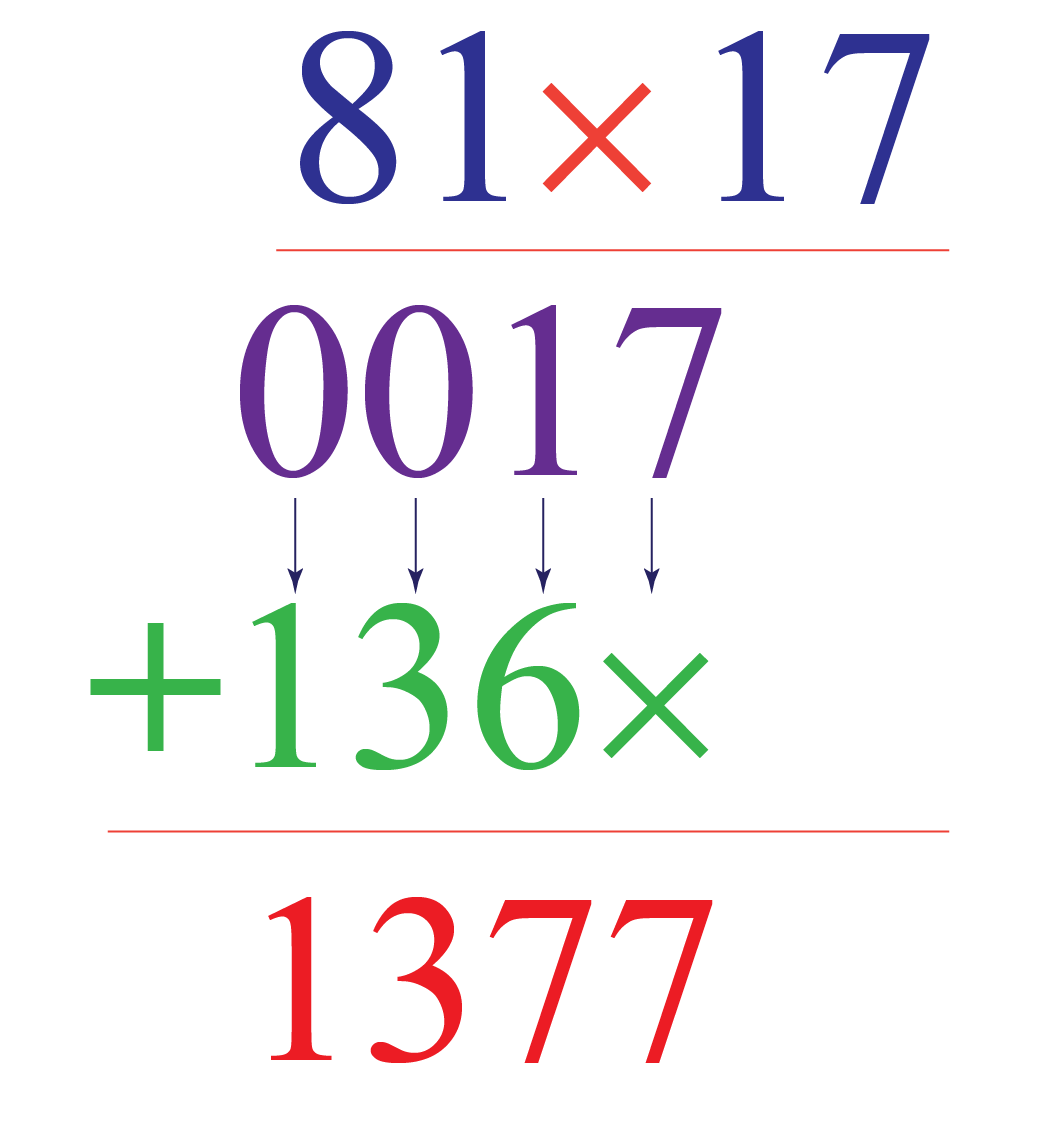### Example

Multiply: $$39 \times 121$$

### Solution

While performing the multiplication, keep the smaller number to the right-hand side.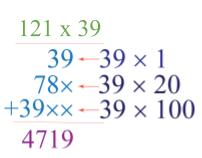Think Tank
• What is the multiplication of 111 and 111? What if we change these numbers to 1111 and 1111 or 11111 and 11111, can we find any pattern for the multiplication of these numbers? Think about it.
• Is the multiplication of the first 10 odd numbers is greater than the multiplication of the first 10 even numbers? Think about it.

## What Is the Column Method of Multiplication?

The column method of multiplication is almost as same as the long multiplication method, the only difference is here in the long multiplication method we perform multiplication horizontally, and in the column method of multiplication, we perform multiplication vertically.

### Example

Multiply: $$52 \times 11$$ by column method.

### Solution

Step 1: Arrange the numbers in a vertical manner as shown below.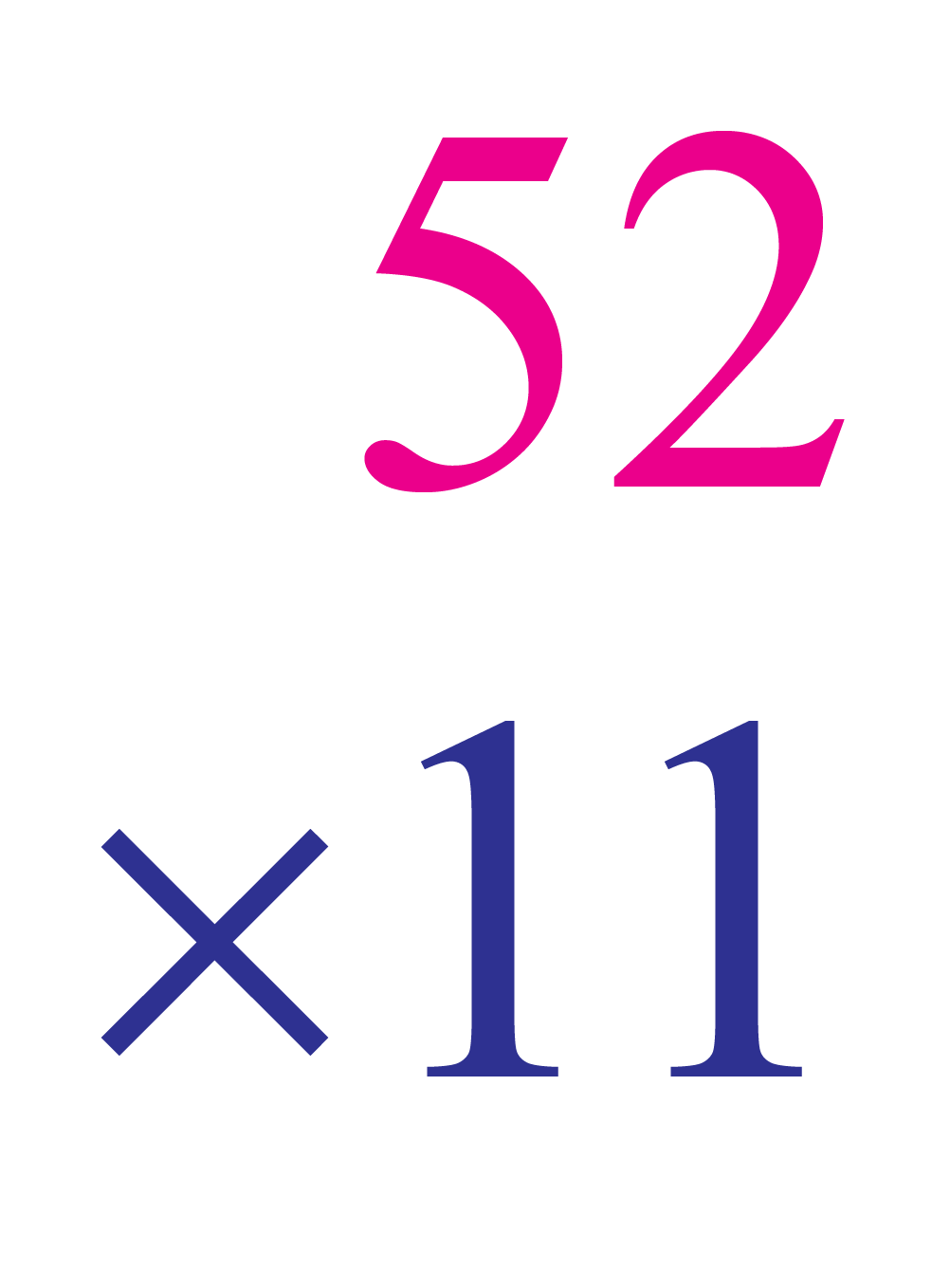Step 2: First multiply 52 with 1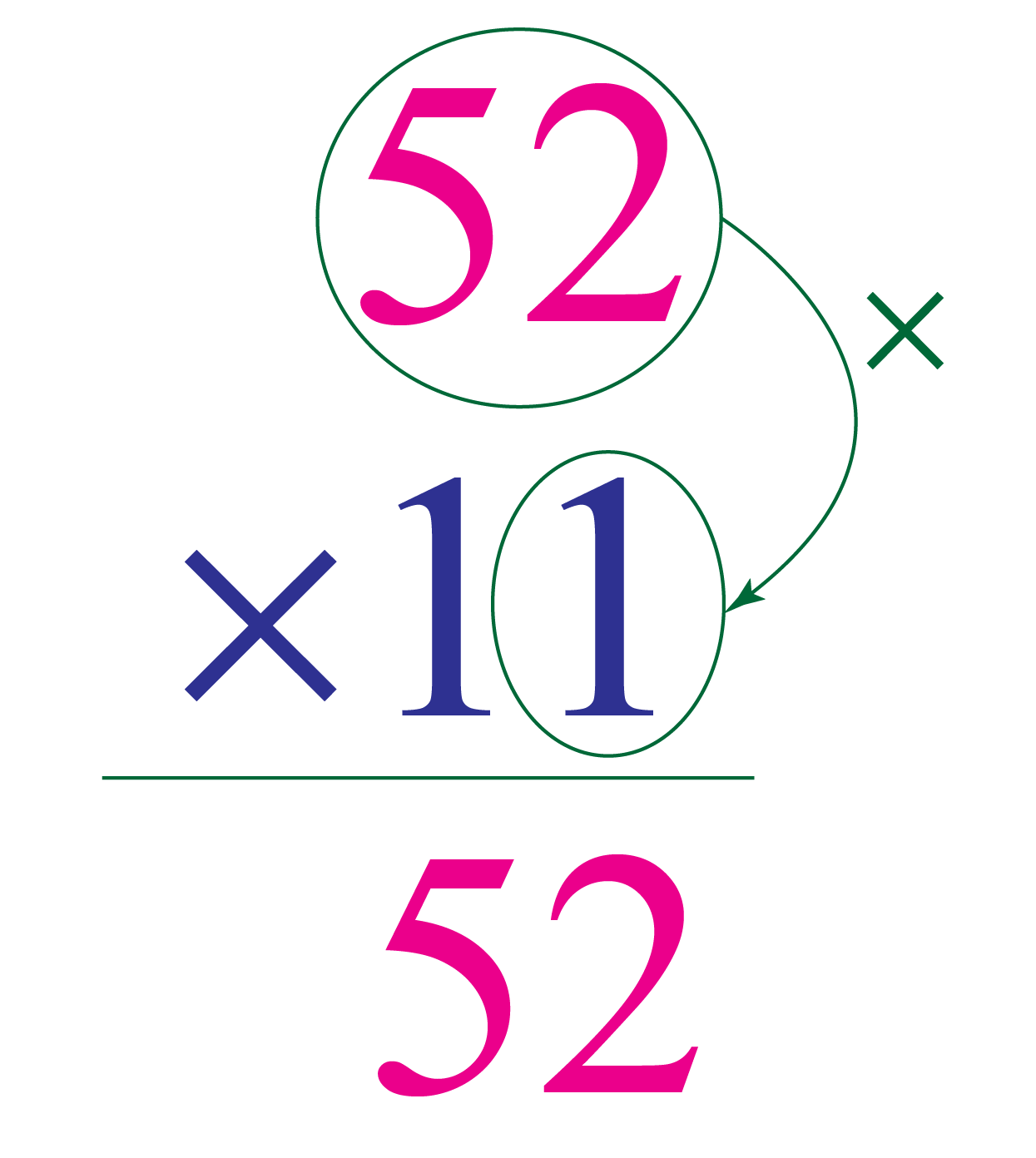Step 3: Now multiply 52 with the 1 at the tenth place, here we are actually multiplying 52 with 10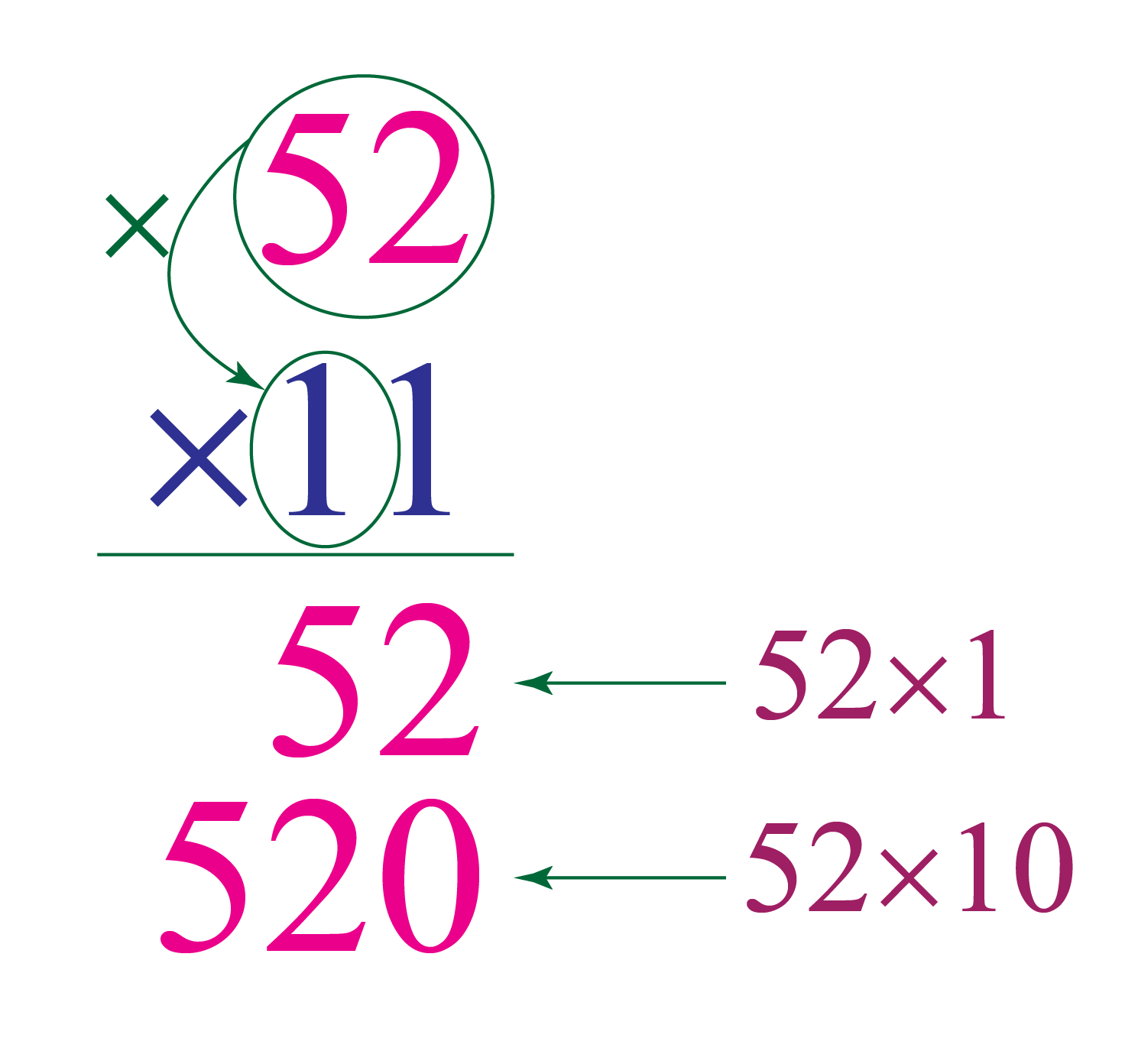Step 4: Now add 52 and 520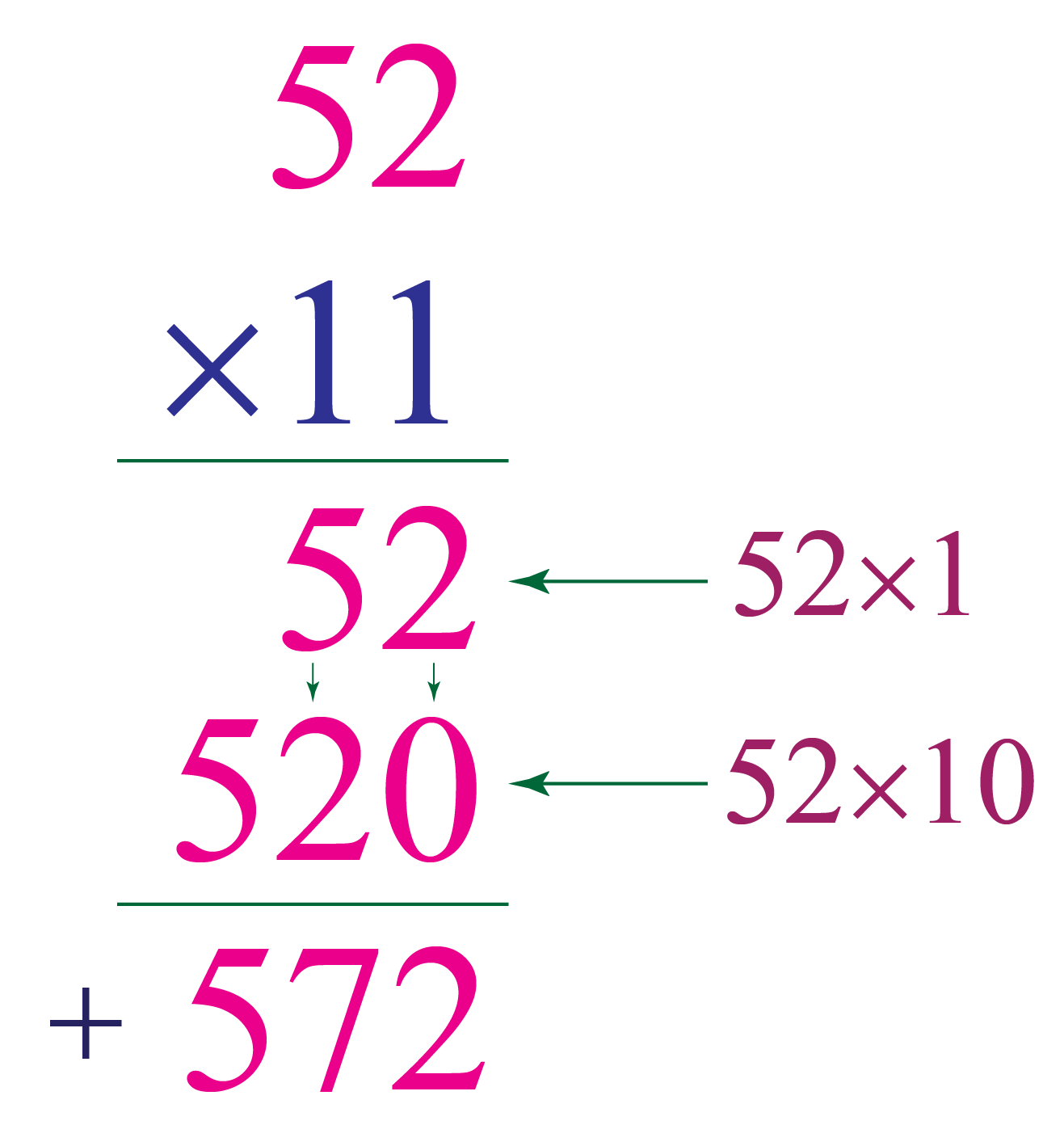So, $$52 \times 11 = 572$$

## Long Multiplication With Decimals

Let's take a few examples of long multiplication with decimal numbers.

### Example

Multiply: $$4.1 \times 2.7$$

### Solution

While performing the multiplication, keep the smaller number to the right-hand side.

Step 1: Remove the decimal and convert the decimal number into a fraction.

$4.1 \times 2.7 \\[0.2cm] \dfrac{41}{10} \times \dfrac{27}{10}$

Step 2: Now keep the 10s in the denominator.

$\dfrac{41}{10} \times \dfrac{27}{10}\\[0.2cm] \dfrac{41 \times 27}{10 \times 10}$

Step 3: Perform the long multiplication on numerators and keep the denominator aside for a while.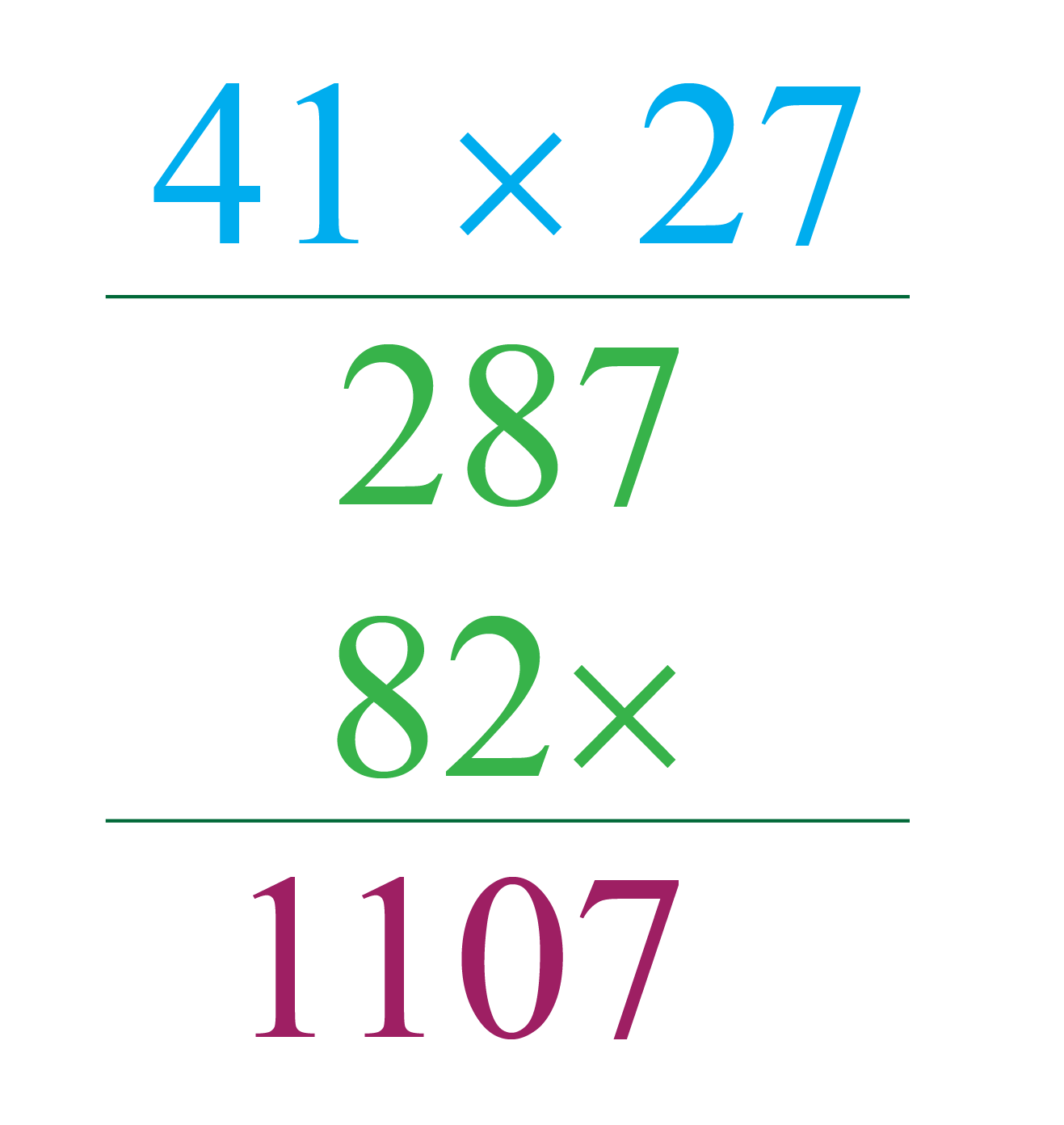Step 4: Now divide the result of the multiplication by the denominator that we kept aside.

$\dfrac{1107}{10 \times 10} \\[0.2cm] \dfrac{1107}{100} \\[0.2cm] \text{11.07}$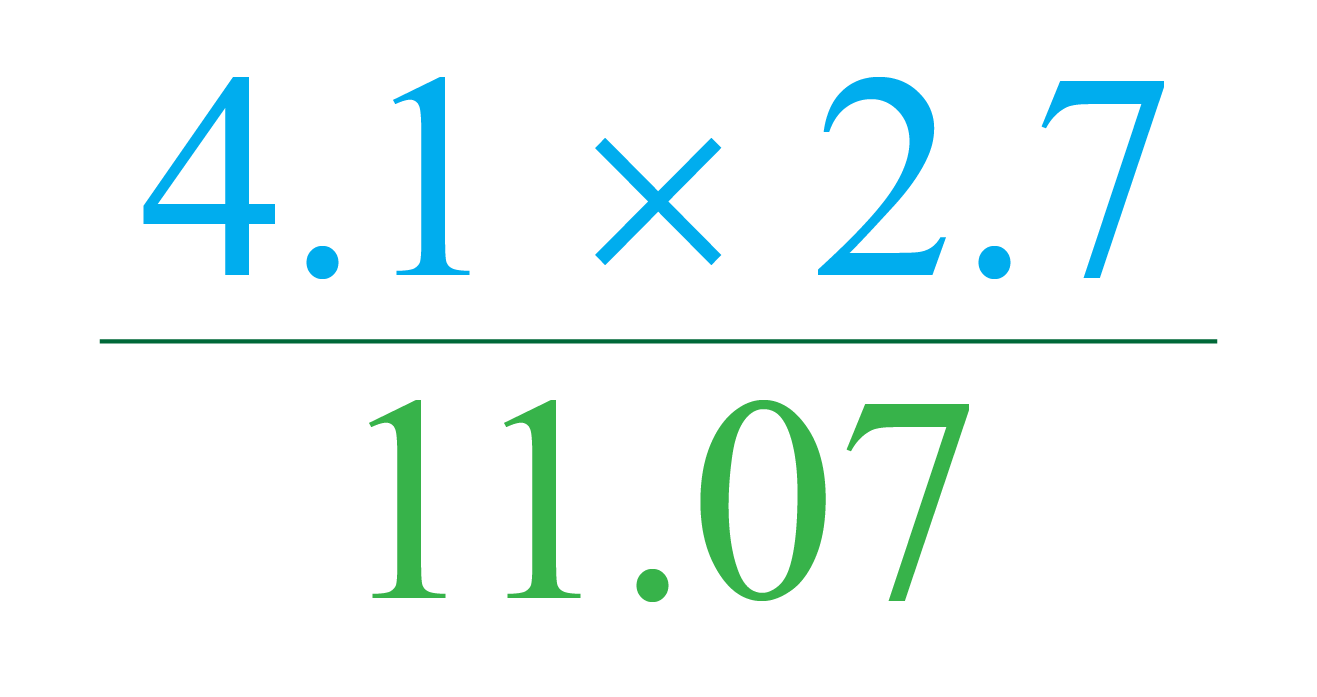## Long Multiplication With Negative Numbers

The long multiplication on negative numbers follows the same rule as positive numbers, the only difference is the sign convention.

We have to remember the sign conventions while multiplying the numbers.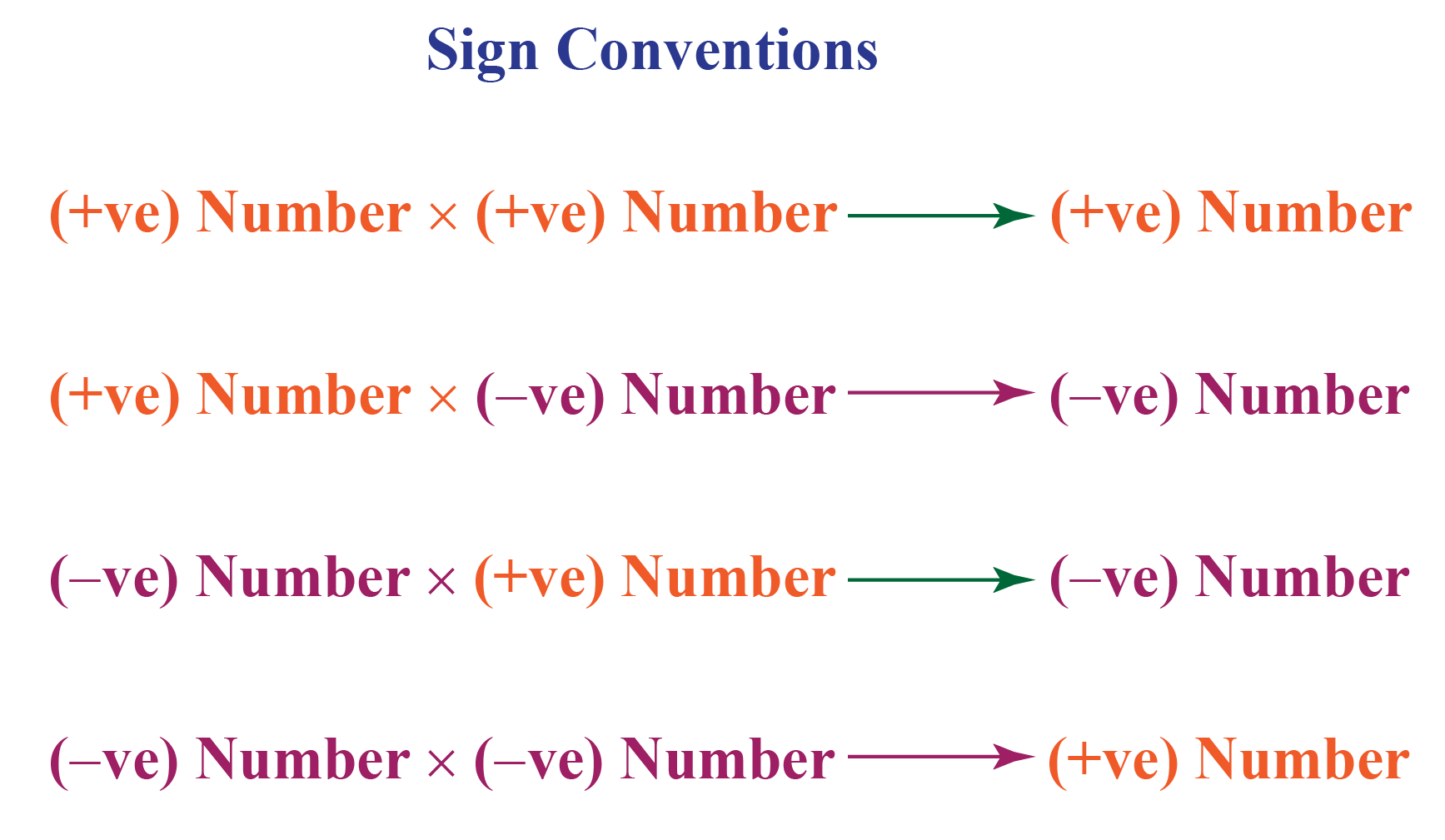## Solved Examples on Long Multiplication

 Example 1

An NGO creating wall paintings on all the metro stations in a city, if a city has a total of 23 metro stations and the NGO decided to run the campaign in 19 such cities. How many metro stations can they cover?

Solution

Given:

Metro stations in a city = 23

Number of cities = 19

Total metro stations covered by the NGO will be-

$23 \times 19 \\[0.2cm] \text{________________}\\[0.2cm] \text{ }\text{ }\text{ } 2 \text{ } 0 \text{ } 7 \\[0.2cm] +\text{ } 2 \text{ } 3 \text{ } \text{x} \\[0.2cm] \text{________________}\\[0.2cm] 437$

 $$\therefore$$ NGO will paint total 437 metro stations.
 Example 2

Help Paul to perform the multiplication $$101 \times 34$$

Solution

We can solve $$101 \times 34$$ by the long multiplication method.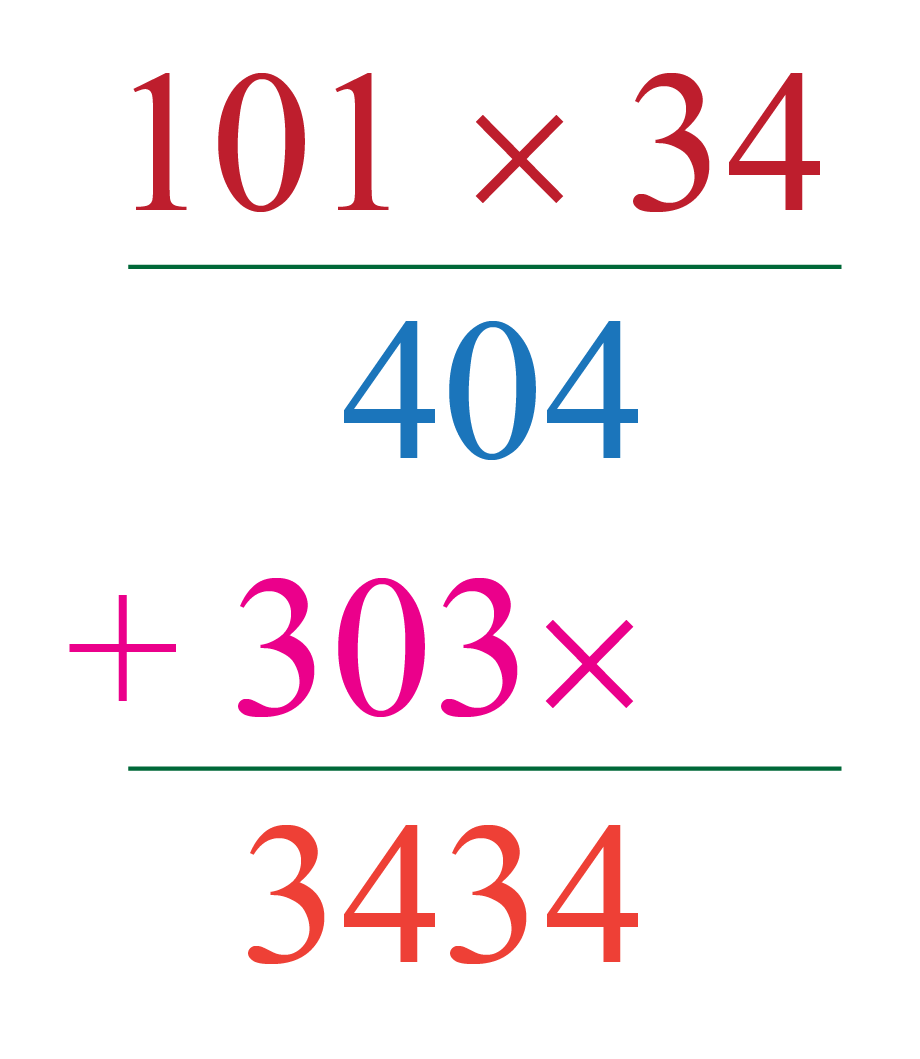$$\therefore 101 \times 34 = 3434$$
 Example 3

Help Tim to multiply $$3214 \times 4561$$ by column method of multiplication.

Solution

We can solve $$3214 \times 4561$$ by the column method of multiplication.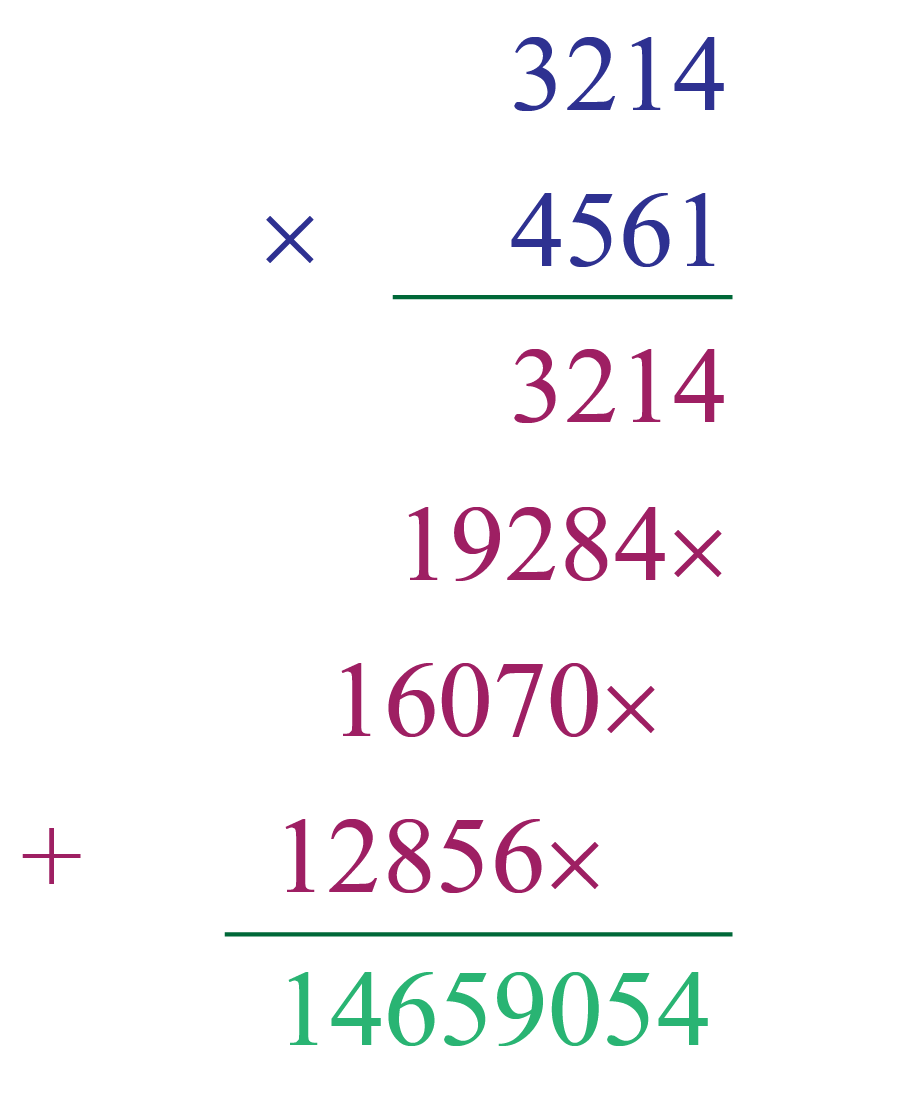$$\therefore 14659054$$

## Interactive Questions on Long Multiplication

Here are a few activities for you to practice. Select/Type your answer and click the "Check Answer" button to see the result.

## Let's Summarize

This mini-lesson introduced you to the fascinating concept of long multiplication. The math journey around long multiplication starts with what a student already knows, and goes on to creatively crafting a fresh concept in the young minds. Done in a way that is not only relatable and easy to grasp, but also will stay with them forever. Here lies the magic with Cuemath.

At Cuemath, our team of math experts is dedicated to making learning fun for our favorite readers, the students! Through an interactive and engaging learning-teaching-learning approach, the teachers explore all angles of a topic. Be it simulations, online classes, doubt sessions, or any other form of relation, it’s the logical thinking and smart learning approach that we at Cuemath believe in.

## FAQs on Long Multiplication

### 1. Multiply using Long multiplication with Step by step method.

Let's take an example

Multiply: $$13 \times 12$$

$$13 \times 12$$

Step 1: First multiply 12 with 3

Step 2: Now, multiply 12 with 1, and put a cross sign just below 6 (the unit place of the number 36), this cross sign represents a 0.

Step 3: Write zeros before the number 36 so that it will cover till the number 1 of 12, write the numbers just below another number so that the addition becomes easy.

Step 4: Add these two numbers 36 and 120

$13 \times 12 \\[0.2cm] \text{________________}\\[0.2cm] \text{ }\text{ }\text{ } 3 \text{ } 6 \\[0.2cm] +\text{ } 1 \text{ } 2 \text{ } \text{X} \\[0.2cm] \text{________________}\\[0.2cm] 156$

More Important Topics
Numbers
Algebra
Geometry
Measurement
Money
Data
Trigonometry
Calculus